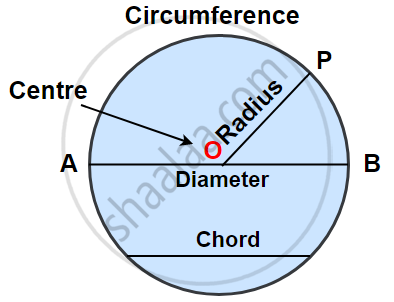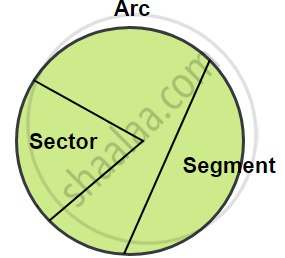Share

# Concept of Circles

Course

## Circles:

• The collection of all the points in a plane, which are at a fixed distance from a fixed point in the plane, is called a circle.• The fixed point is called the centre of the circle.

• The fixed distance is called the radius of the circle.
The line segment joining the centre and any point on the circle is also called a radius of the circle. That is, ‘radius’ is used in two senses in the sense of a line segment and also in the sense of its length.

• The Perimeter of the circle is called the circumference.

• The line segment, joining any two points on the circumference of the circle, is called a chord.

• A chord, which passes through the centre of the circle is called the diameter, and is the largest chord of the circle which is equal to two times the radius.•  A sector is a region in the interior of a circle enclosed by an arc on one side and a pair of radii on the other two sides.
• A segment of a circle is a region in the interior of the circle enclosed by an arc and a chord.

• A diameter of a circle divides it into two equal parts; each part is a semi-circle. A semi-circle is half of a circle, with the endpoints of diameter as part of the boundary.

### Shaalaa.com

Circles [00:15:54]
S
Circles
0%